Courses

# Very Short Answers Type Questions- Statistics Class 9 Notes | EduRev

## Mathematics (Maths) Class 9

Created by: Full Circle

## Class 9 : Very Short Answers Type Questions- Statistics Class 9 Notes | EduRev

The document Very Short Answers Type Questions- Statistics Class 9 Notes | EduRev is a part of the Class 9 Course Mathematics (Maths) Class 9.
All you need of Class 9 at this link: Class 9

Question 1. The marks obtained by 17 students in a mathematics test (out of 100) are given below:
48, 66, 68, 49, 91, 72, 64, 46, 90, 79, 76, 82, 65, 96, 100, 82, 100
Find the range of the data.
Solution:
Lowest observation = 46
Highest observation = 100
∴ Range = 100 –46 = 54

Question 2. The class-mark of the class 130 – 150 is:
Solution: Class-mark =  ((130+150)/2)
= (280/2)
= 140

Question 3. The median of the following numbers arranged in descending order is 25. Find the value of
x: 40, 38, 35, 2x + 10, 2x + 1, 15, 11, 8, 5
Solution:
Number of observations = 9

∴ The median is the  ((n+1)/2)th  term i.e. ((9+1)/2) th or the 5th term.
⇒ 2x + 1 = 25
⇒ x= (25-1)/2= 12

Question 4. If the mean of 6, x, 4, and 12 is 8, then find the value of x.
Solution: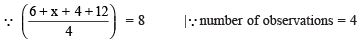∴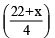= 8 or 22 + x = 32
or  x = 32 – 22 = 10

Question 5. Find the range of the data 9, 7, 5, 7, 9, 9, 6, 18, 9 and 8.
Solution:
Highest data = 18
Lowest data = 5
⇒ Range = 18 – 5 = 13

Question 6. Calculate the median of : 152, 155, 160, 144, 145, 148, 147, 149, 150
Solution
:  Writing the given data in ascending order, we get: 144, 145, 147, 148, 149, 150, 152, 155, 160
Here, n = 9

∴ Median =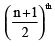term =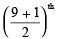term = 5th term

⇒ Median = 149

Question 7. What is the median of 70, 40, 50, 100, 75, 75, 65 and 95?
Solution:
In ascending order, the given data is:
40, 50, 65, 70, 75, 75, 95, 100
Here, n = 8 (Even number)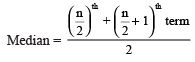⇒ Median =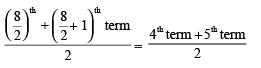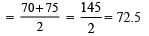## Mathematics (Maths) Class 9

192 videos|230 docs|82 tests

,

,

,

,

,

,

,

,

,

,

,

,

,

,

,

,

,

,

,

,

,

;